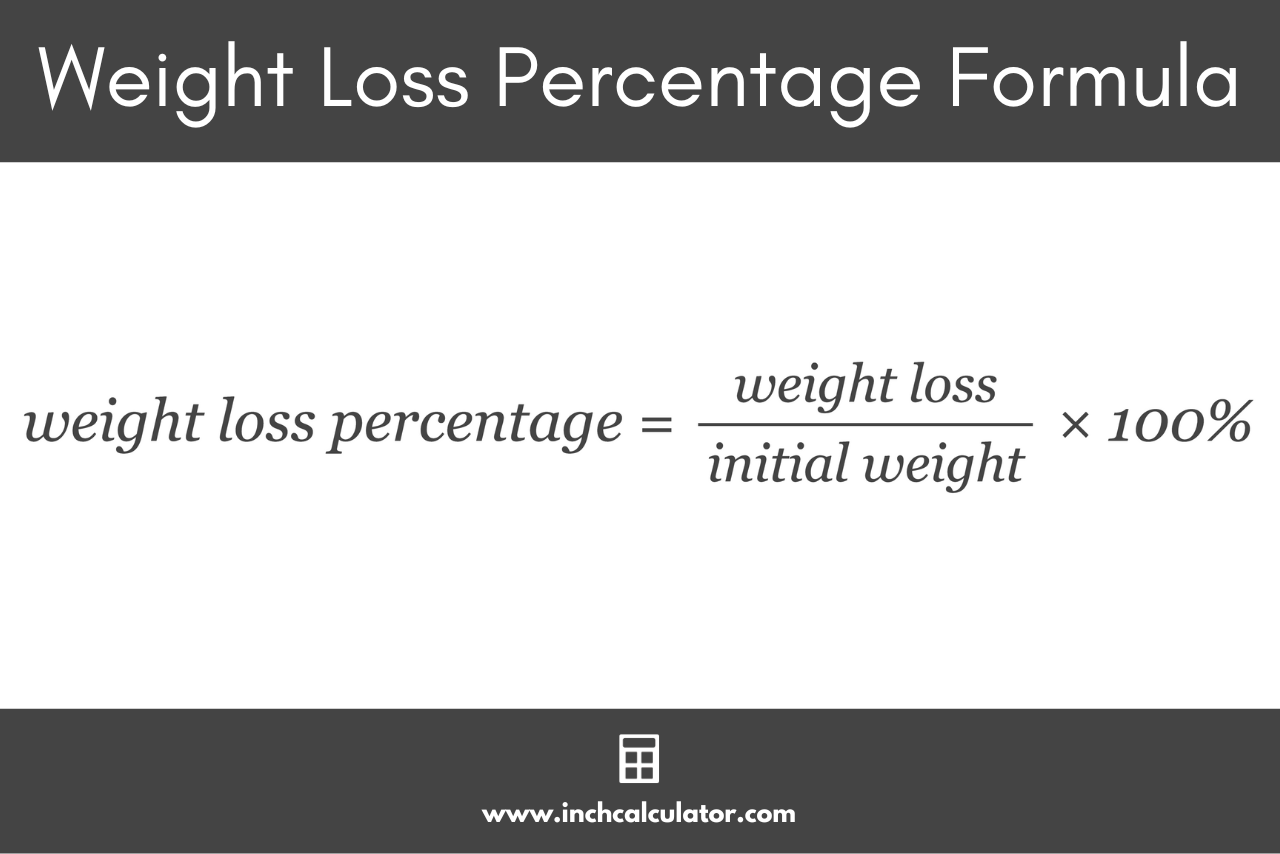# Weight Loss Percentage Calculator

Use our weight loss percentage to track your drop in weight in the form of a percentage.

## Weight Loss Percentage:

%

### Total Weight Loss

Learn how we calculated this below

## How to Calculate a Weight Loss Percentage

Whether you’re participating in a weight loss competition, setting personal fitness goals, or tracking your progress over time, it’s often useful to express weight loss as a percentage. This is a standardized measure that helps compare progress irrespective of initial body weight.

Expressing weight loss as a percentage offers several advantages:

• Standardization: It allows individuals of different weights to compare their progress on a level playing field.
• Motivation: Seeing tangible progress, denoted as a percentage, can be a morale booster.
• Setting Realistic Goals: Knowing average percentages of weight loss can help in setting achievable targets.

You can calculate a weight loss percentage using the percent decrease formula.

### Weight Loss Percentage Formula

Using the percent decrease formula, the formula to calculate a weight loss percentage is:

weight loss percentage = weight loss / initial weight × 100%

Thus, the weight loss percentage is equal to the total weight lost divided by the initial weight, multiplied by 100%. You can calculate your total weight loss by subtracting your current weight from your starting weight.For example, let’s say someone started at 200 pounds and has dropped their weight to 180 pounds. You can calculate their weight loss as a percentage using the formula above.

Start by finding the amount of weight they lost.

200 lbs – 180 lbs = 20 lbs lost

Then, divide the weight loss by the initial weight.

20 lbs / 200 lbs × 100% = 10%

So, in this example, this person lost 10% of their initial body weight.

If you’re trying to lose weight, we suggest using our weight loss calculator to calculate how many calories you should consume to lose weight.